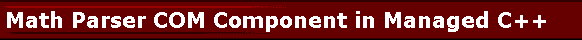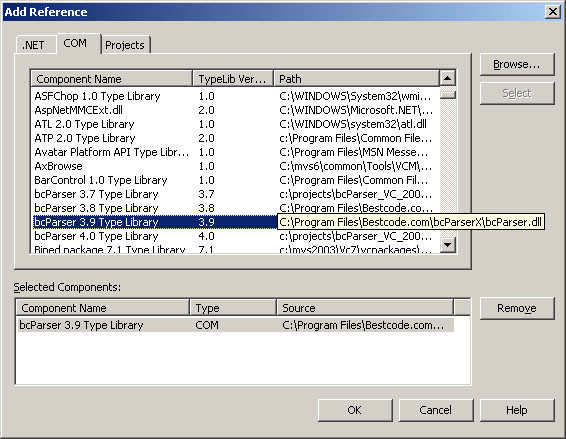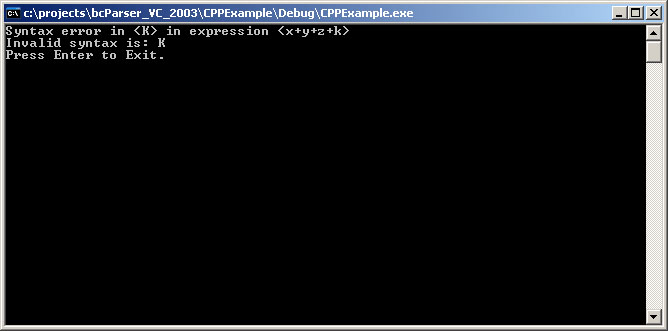# How to Parse Math Expressions in Managed C++

bcParserX COM Component can be used from managed code as well as native code. Here we will show a simple Managed C++ (CPlusPlus) console application to demonstrate simple the steps required to get math expression parser working.

The sample starts with creating a instance of the COM object to use. Then we set the expression to parse. Then we assign predefined variables X and Y. And we create a new variable called Z. Notice that this sample intentionally forgets to create variable K. Then we evaluate the expression and display the result. When we execute this code, we get an exception that says expression is not valid. In the catch block, we display this error. Then we also display the invalid portion of the expression which says K. This functionality is useful for some applications that wish to recover from certain errors by defining K and continue.

Step 1: To be able to use the COM component, you need to add a reference to it in your project. Notice the BCPARSERLib on the right under References. Right click references to add it from installed COM Components List.Step 2: Type the Code: (Make sure you include necessary namespaces as seen below: Interop, InteropServices)

 //This is an example application that demonstrates the use of //Bestcode.com Math Parser COM Component in managed C++ application. #include "stdafx.h" #using using namespace System; using namespace Interop::BCPARSERLib; //to use Math Parser COM Component. using namespace System::Runtime::InteropServices; //COMException int _tmain() {         IParser *p = new ParserClass();         try         {                 //set expression:                 p->Expression = "x+y+z+k"; //notice that k is not valid.                 p->set_X(5); //assign predefined var X.                 p->set_Y(6); //assign predefined var Y.                 p->set_Variable("Z", 1); //create new var Z.                 double value = p->Evaluate(); //get result.                 Console::WriteLine(S"Answer={0}", __box(value));         }         catch(COMException *ex)         {                 Console::WriteLine(ex->Message);                 Console::WriteLine(S"Invalid syntax is: {0}",                         p->GetInvalidPortionOfExpression());         }         Console::WriteLine("Press Enter to Exit.");         Console::ReadLine();         return 0; }

As simple as that! And now you can have powerful math expression parsing capabilities in your application with few lines of code too!

Step 3: Execute! When you run this application, output is:## Adding User Defined Functions to Math Parser COM Component

Below example defines user functions that takes 1, 2, 3, or more parameters, and registers their function address with the Math Parser COM Component. Note that this apporach uses raw function pointers. It is also possible to use COM events which is explained in C# Example. However, for C++ applications, using raw pointers like this gives better performance.

 //This is an example application that demonstrates the use of //Bestcode.com Math Parser COM Component in managed C++ application. #include "stdafx.h" #using using namespace System; using namespace Interop::BCPARSERLib; //to use Math Parser COM Component. using namespace System::Runtime::InteropServices; //COMException //User defined function that takes one parameter: long double __stdcall FuncOne(const long double p){         return p; } //User defined function that takes two parameters: long double __stdcall FuncTwo(const long double p1, const long double p2){         return p1+p2; } //User defined function that takes 3 parameters: long double __stdcall FuncThree(const long double p1, const long double p2, const long double p3){         return p1+p2+p3; } //User defined function that takes N parameters: long double __stdcall FuncMultAll(const long double p[], const int paramCount){         long double val=1;         for(int i=0; iCreateOneParamFuncwAddress("ONE", (int)FuncOne); //pass the function address as integer.                 p->CreateTwoParamFuncwAddress("TWO", (int)FuncTwo);                 p->Create3ParamFuncwAddress("THREE", (int)FuncThree);                 p->CreateNParamFuncwAddress("NP", (int)FuncMultAll);                 //set expression:                 //p->Expression = "x+y+z+k"; //notice that k is not valid.                 p->Expression = "ONE(1)+TWO(2,3)+THREE(1,2,3)+NP(1,2,3,4,5)";                 p->set_X(5); //assign predefined var X.                 p->set_Y(6); //assign predefined var Y.                 p->set_Variable("Z", 1); //create new var Z.                 double value = p->Evaluate(); //get result.                 Console::WriteLine(S"Answer={0}", __box(value));         }         catch(COMException *ex)         {                 Console::WriteLine(ex->Message);                 Console::WriteLine(S"Invalid syntax is: {0}",                         p->GetInvalidPortionOfExpression());         }         Console::WriteLine("Press Enter to Exit.");         Console::ReadLine();         return 0; }

Enjoy! Best Regards!

webmaster@gobestcode.com